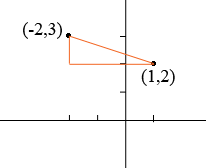SEARCH HOMEMath Central Quandaries & QueriesQuestion from Trent, a student: write the equation of circle B with center B(-2,3) that passes through (1,2)Hi Trent,

I would first draw a diagram. I added three line segments to form a right triangle.Use either the distance formula or Pythagoras Theorem to find the distance $r$ between the two points. Since one point is the center of the circle and the other is on the circle $r$ is the radius of the circle.

Let $(x, y)$ be some point in the plane. $(x, y)$ is on the circle if and only if the distance from $(-2, 3)$ to $(x, y)$ is $r.$ Use the distance formula to find the distance from and set it equal to $r$ to form an equation. Square both sides and simplify.

PennyMath Central is supported by the University of Regina and the Imperial Oil Foundation.